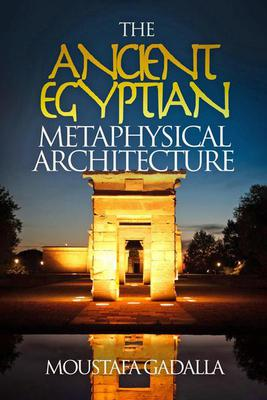# Squaring The Circle

## Squaring The Circle

### The Manifestation Of Creation

“Squaring the circle” for the Ancient Egyptians represented the realization of creation—the transformation process of the concept of creation into its actual manifestation.

Such transformation is reflected and evident in all Ancient Egyptian “mathematical” papyri. In all these papyri, the area of a circle was obtained by squaring the circle. The diameter was always represented as 9 cubits. The Ancient Egyptian papyri equates the 9-cubit diameter circle to a square with the sides of 8 cubits.The number 9, as the diameter, represents the Ennead, the group of 9 neteru (gods, goddesses) who produced the ingredients of creation. The 9 are all aspects of Re, the primeval cosmic creative force whose symbol is/was the circle.

8 corresponds to the physical world as we experience it. 8 is the number of Thoth, and at Khmunu (Hermopolis), Thoth is known as the Master of the City of Eight.

Musically, the ratio 8:9 is the Perfect Tone. The 8:9 ratio is present in Ancient Egyptian works, such as the proportion of the inner chamber of the top sanctuary at Luxor Temple.

The underlying metaphysical patterns of the manifested universe are represented in the relationship of squaring the circle (Re and Thoth—conceived and manifested).

Thoth transformed the creation concept (symbolized in a circle) into a physical and metaphysical reality. Such transformation is reflected in the Ancient Egyptian process of “squaring the circle”.

The area of a circle with 9 cubits as its diameter = 63.61725.

The area of the squared circle with 8 cubits as its side = 64.

The difference = 64 – 63.61725 = 0.38.

Such a difference = 0.6%, which reflects the Ancient Egyptian consideration of a slight deviation from perfection in the manifested world.

A good example of this slight imperfection is the orbit of the Earth around the sun, which follows an elliptical shape and not a perfect circle.

Musically, the ratio 8:9 is the Perfect Tone.

The ratio 8:9 = 2 to its 3rd power and 3 to its 2nd power. This is the perfect relationship between the reciprocals of 2 and 3 to their reciprocal powers of 3 and 2. The numbers 2 and 3 are the two primary cosmic numbers, as will be discussed in Chapter 9 of this book.

The walls of the Egyptian temple were covered with animated images—including hieroglyphs—to facilitate the communication between the above and the below.

The Ancient Egyptian framework was usually a square, representing the manifested world (squaring of the circle). Additionally, the square grid itself had symbolic meaning for the manifested world, which also made it easy to construct the root rectangles of 2, 3, and 5, on/by the square(s) background. The corners of squares and root rectangles were defined by notches along the perimeter or carefully defined by incised lines.

[An excerpt from The Ancient Egyptian Metaphysical Architecture by Moustafa Gadalla]
https://egyptianwisdomcenter.org/product/the-ancient-egyptian-metaphysical-architecture/https://egyptianwisdomcenter.org/product/the-ancient-egyptian-metaphysical-architecture/